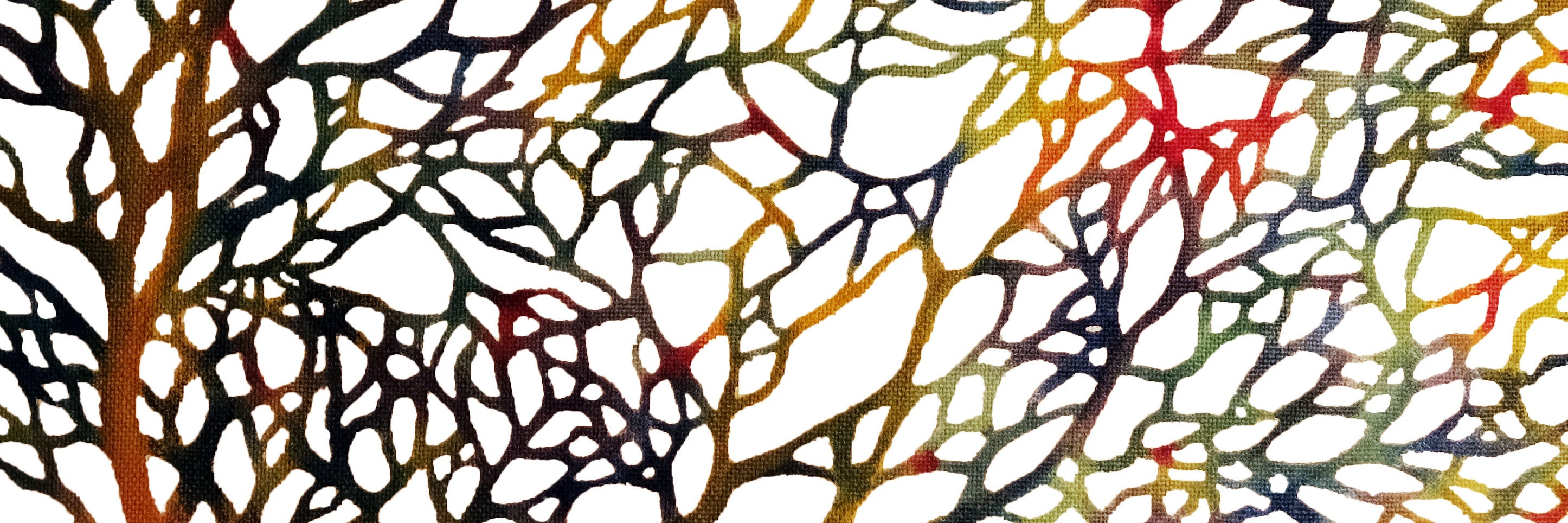•## Dendritic computers: When 1 + 1 = 3

The human brain is jampacked with neurons – 86 billion of them – connected in a vast, complex network that controls how we move, think, talk and m...

• ## Mini Course 7

In linear algebra, eigendecomposition or sometimes spectral decomposition is the factorization of a matrix into a canonical form, whereby the matrix i...

• ## Mini Course 10

In linear algebra, eigendecomposition or sometimes spectral decomposition is the factorization of a matrix into a canonical form, whereby the matrix i...

• ## Mini Course 9

In linear algebra, eigendecomposition or sometimes spectral decomposition is the factorization of a matrix into a canonical form, whereby the matrix i...

• ## Mini Course 8

In linear algebra, eigendecomposition or sometimes spectral decomposition is the factorization of a matrix into a canonical form, whereby the matrix i...

• ## Mini Course 6

In linear algebra, eigendecomposition or sometimes spectral decomposition is the factorization of a matrix into a canonical form, whereby the matrix i...

• ## Mini Course 5

In linear algebra, eigendecomposition or sometimes spectral decomposition is the factorization of a matrix into a canonical form, whereby the matrix i...

• ## Mini Course 4

In linear algebra, eigendecomposition or sometimes spectral decomposition is the factorization of a matrix into a canonical form, whereby the matrix i...

• ## Mini Course 2

In linear algebra, eigendecomposition or sometimes spectral decomposition is the factorization of a matrix into a canonical form, whereby the matrix i...

• ## Mini Course 11

In linear algebra, eigendecomposition or sometimes spectral decomposition is the factorization of a matrix into a canonical form, whereby the matrix i...

Search found 1842 items